TUTORIALS
TUTORIALS HOME

GENERAL MATH
NUMBER SETS
ABSOLUTE VALUE & INEQUALITIES
SETS & INTERVALS
FRACTIONS
POLYNOMIALS
LINEAR EQUATIONS
GEOMETRY
FINITE SERIES
TRIGONOMETRY
EXPONENTS
LOGARITHMS
INDUCTION

CALCULUS
LIMITS
DERIVATIVES
RELATED RATES & OPTIMIZATION
CURVE SKETCHING
INTEGRALS
AREA & VOLUME
INVERSE FUNCTIONS

MAIN
HOME
TESTS
TUTORIALS
SAMPLE PROBLEMS
COMMON MISTAKES
STUDY TIPS
GLOSSARY
CALCULUS APPLICATIONS
MATH HUMOUR

### LINEAR EQUATIONS TUTORIAL

A linear equation is an equation whose graph is a straight line. A linear equation in one variable is an equation that simlpy involves x. There are no terms involving x2, x3, x1/2 etc. Each term has a degree of at most 1. All operations, such as addition or multiplication, involve only x and numerical constants. 3x + 4 = 5 is an example of a linear equation. 2(x+1) = 6(x-4) is also a linear equation. These equations can be solved very easily by performing algebraic operations to the equation to isolate x.

A linear equation in two variables is, as the name suggests, an equation that involves 2 variables. The standard form of this type of equation is Ax + By = C, where A,B and C are real numbers. For example, 3x + y = 7 is a linear equation in two variables. y = 2x + 1/3 is also an example, since it can be rewritten as 2x - y = -1/3 ( or equivalently 6x - 3y = -1 ).

# Slope-Intercept Form

Linear equations in two variables can also be expressed in the slope-intercept form y = mx + b.

The slope of a line, represented by the variable m, is defined as the ratio of change in values of y to change in value of x. The slope is also known as rise over run. For any two points (x1 ,y1), (x2 ,y2) on a line L, the formula for calculating the slope of L is: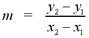The y-intercept, denoted b, is the point (0,b), where the line intercepts the y-axis.

There are two variations of the slope-intercept form of a line. The first is called the point-slope form of the equation of a line. This formula is used when the slope and a single point on the line are given in the problem. The formula for the point-slope form is: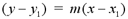The other variation is the two-point form of the equation of a line. This is simply the point-slope formula, with the slope formula substituted for m. This formula should be used when two points on a line are given in the problem. the formula for the two-point form is: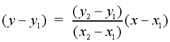The vertical line x = a represents a special case of the slope-intercept form of a line. Since (x2 - x1) = 0, the slope of the line is m = (y2 - y1)/0. Since division by 0 is undefined, the slope of the line is undefined. The line is also expressed in terms of x, rather than y. Due to these properties, vertical lines cannot be expressed using the slope-intercept form.

# Examples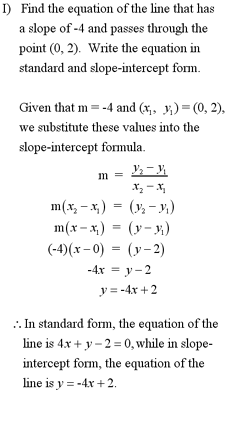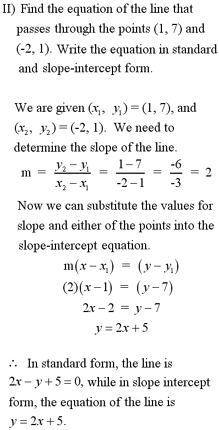Note: For another example of finding the equation of a line, see question #1 in the Additional Examples section at the bottom of the page.

# Line Theorems

Two lines are parallel if they have equal slopes. Parallel lines never cross each other. The distance between two parallel lines is always the same for every point along the lines.

Two lines are perpendicular, meaning their angle of intersection is 90°, if their slopes are negative reciprocals of each other. For lines L1 and L2 with slopes m1 and m2, respectively,The midpoint of a line segment joining any two points (x1, y1) and (x2, y2) is the point given by: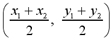The distance, D, between any two points (x1, y1) and (x2, y2) is given by: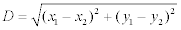# Graphing Linear Equations

Linear equations in one variable have graphs of either horizontal or vertical lines, depending on which co-ordinate plane variable is used. For equations of the form x = a, the graph is a vertical line, intersecting the x-axis at (a,0). For equations of the form y = b, the graph is a horizontal line intersecting the y-axis at (0,b).

Linear equations are simply straight lines. If you can determine two points that lie on a line, then the graph is the line that connects those two points. This is sometimes referred to as the two point method of graphing linear equations.

The most common points to determine from the equation are the x and y intercepts, (x,0) and (0,y), respectively. These points can be determined by substituting x = 0 and y = 0 into the equation and finding the corresponding y and x values.

The slope-intercept method can also be used to graph linear equations. The equation should be written in slope-intercept form so the slope and y-intercept can be easily identified. After plotting the y-intercept on the graph, a second point can be obtained using the ratio of the slope. The graph is simply the line that connects these points.

# Examples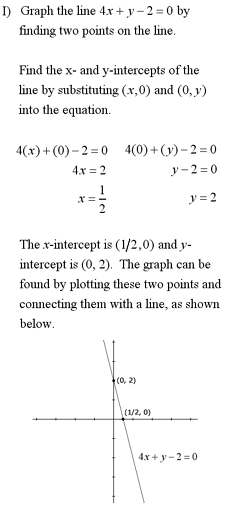Note: For another example of graphing a linear equation, see question #1 in the Additional Examples section below.

1 | Find and graph the equation of the line

| Top of Page |

COURSE HOMEPAGES
MATH 1036
MATH 1037

FACULTY HOMEPAGES
Alex Karassev
Ted Chase